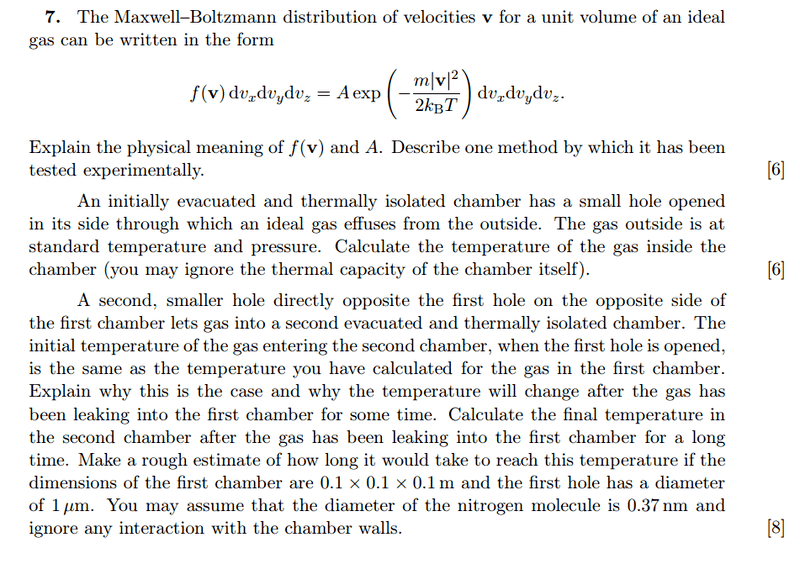# Effusion through hole of box

## Homework StatementPart (a): Explain what A and f means. Describe an experiment that shows this relation is true.
Part (b):Find the temperature of vessel 1.
Part (c): Why is temperature of vessel initially the same as vessel 1? Calculate the final temperature of vessel 2. Find the time taken for vessel 2 to reach this temperature.

## The Attempt at a Solution

Part (b)
Letting ##T_0 = 300 K## be room temperature, and ##\alpha = \frac{m}{2kT}##,
the speed distribution of effusing molecules from 1 into 2 is:

$$g_{(v)} = 2 \alpha^2 v^3 \space exp\left(-\alpha v^2\right)$$

The average energy of these particles is:

$$\langle E'\rangle = \frac{1}{2}m \int_0^{\infty} 2\alpha^2 v^5 \space exp\left(-\alpha v^2\right)$$
$$= 2kT_0$$

Now, in general the average KE of a system with temperature ##T## is ##\frac{3}{2}kT##.

$$\langle E' \rangle = \frac{3}{2}k\left(\frac{4}{3}T_0\right)$$
$$= \frac{3}{2}kT_1$$

This implies that temperature of box 1 is ##T_1 = \frac{4}{3}T_0 = 400 K##.

Part (c)
In the beginning, the average KE of molecules entering box 2 should be higher than the average KE of particles in box 1, since particles effusing have higher KE? I'm not sure why it would be the same.

Now the same as above,
$$T_2 = \frac{4}{3}T_1 = 533 K$$

The flux of particles effusing from box 1 is given by:
$$\phi = \frac{1}{4}n \langle v_1\rangle = \frac{1}{4} \frac{P}{kT} \sqrt{\frac{8kT_1}{\pi m}}$$

Thus number of particles per unit time is ##\phi A = \pi r^2## where ##r^2## is the area of the hole.

Not sure how to find the time taken.

TSny
Homework Helper
Gold Member
Your result for part (b) looks correct for a monatomic gas where KEavg = (3/2)kT. (But note that at the end of the statement of the problem, it mentions nitrogen which is diatomic.)

For part (c), you are looking for the initial temperature in box 2 a short time after hole 1 is opened. Almost all of the molecules that make it through hole 2 initially are those molecules that travel directly from hole 1 through hole 2 without any collisions on the way. See if you can show that the average translational KE of such a molecule is 2kT0.

Last edited:
•1 person
Your result for part (b) looks correct for a monatomic gas where KEavg = (3/2)kT. (But note that at the end of the statement of the problem, it mentions nitrogen which is diatomic.)

For part (c), you are looking for the initial temperature in box 2 a short time after hole 1 is opened. Almost all of the molecules that make it through hole 2 initially are those molecules that travel directly from hole 1 through hole 2 without any collisions on the way. See if you can show that the average translational KE of such a molecule is 2kT0.

Yes, I worked through the question and I got the right answers. Thanks alot!